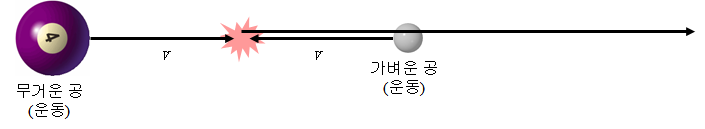# Collision

## Collision of two balls of different mass

#### 1. What happens if you throw a light ball towards a heavy ball?Just like a basketball ball bounces on the surface of large earth, the light ball bounces.
This is easy to understand.

#### 2. When a heavy ball rushes towards a light ballIn this case, you have to think a bit.
It would help if you did a simple coordinate transformation. Remove the speed of v for each ball. Then, you can think that the light ball is approaching while the heavy ball is stopped.
Then, we can think of a light ball hitting a heavy ball surface and then bouncing back.
Then add speed v to each ball. As a result, the light ball bounces with a speed of 2v.

#### 3. When two balls collide with each other at speed vYou can sum both cases. As a result of the crash, the light ball will pop out at speed 3v.

* Special thanks to Ralph Smallberg for the advice.